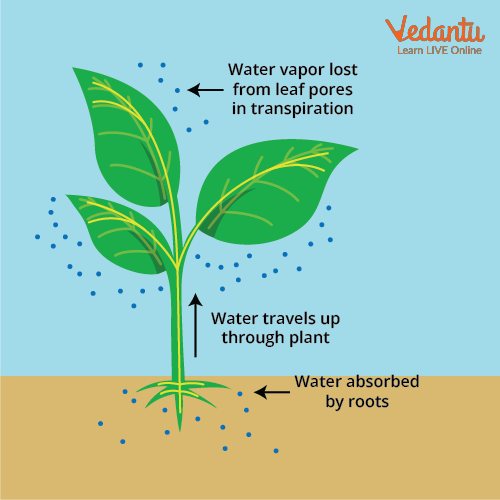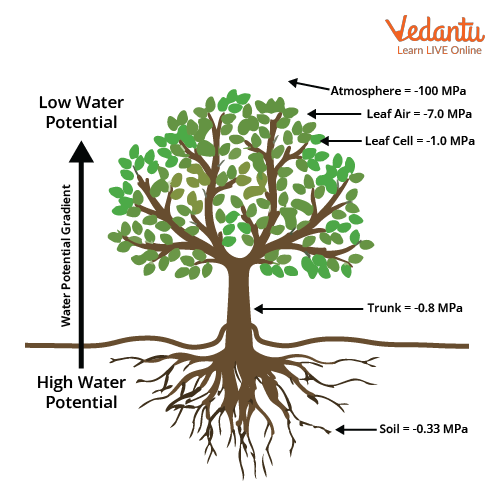Courses
Courses for Kids
Free study material
Free LIVE classes
More

# Water Potential: Important Topic for NEETLIVE
Join Vedantu’s FREE Mastercalss

## What is Water Potential?

Prior to understanding the meaning of water potential, let us understand what is the meaning of potential. It evaluates the inclination of water to move starting with one region then onto the next because of osmosis, gravity, mechanical tension and matrix-like capillary action.

On the other hand, Water potential is the measure of the potential energy of the water; solute potential and pressure potential tend to determine the water potential of a cell. The movement of water is always from the high water potential to the low water potential. Water potential is signified by the Greek image Psi or $\Psi$. Unit of water potential is Pascal (Pa). Water potential of pure water is zero. In addition to some solutes to the pure water, the water potential drops down to negative. Water potential and its components play an important role in the plants.

## Definition of Water Potential

• Water potential is the energy required, per amount of water, to transport a minute amount of water from the sample to a reference pool of pure water.

• It is thus a measure of the free energy of water per unit volume.

• Unit of water potential is Pascal.

• Water potential of pure water is zero because there is a maximum water molecule in pure water.

• A solution will always have a negative water potential.

• If we add solute in pure water, the solution has fewer free water molecules and the concentration of water decreases, so the water potential decreases. In this way, the solution will always have less water potential than zero, i.e. negative.

## Significance of Water Potential

• Water potential is used to describe the direction of movement of water.

• Water molecules will always move from high water potential to low water potential.

• If we immerse a plant cell in a solution which has high water potential than the plant cell, water will start moving into the plant cell from the solution.Movement of water in the plant

## Water Potential in Plants

• Water Potential in plants refers to the movement of water from one part of the plant to another.

• It can never be positive.

• It can be negative but it will never be greater than zero.

• By using this information, we can predict where water will move inside the plant.

• Water potential value will be the greatest negative at the top of the plant because of evaporation.

• Roots have the least negative water potential because they absorb water.

• The soil water potential is -0.33MPa.

• The soil water potential is the least negative.Water potential values in different parts of the plant

## Water Potential Components

• Water potential in plants depends on two major factors-

1. Solute potential $\left( \Psi s \right)$

2. Pressure potential $\left(\Psi _{p}\right)$

## Solute Potential

• The effect of dissolved solutes on water potential is known as Solute potential.

• It is also known as Osmotic Potential.

• Solute potential $\left( \mathbf{\Psi s} \right)$ decreases with increasing the concentration of solute.

•  A decrease in the value of ${\Psi_s}$ causes a reduction in the water potential.

• Its value is negative in plants.

• The negative value is used to indicate that on increasing solute concentration the water potential will decrease.

• A solution which is at atmospheric pressure (water potential) $\Psi w=$ (solute potential) $\Psi s$.

## Pressure Potential

• The pressure which is applied by the protoplasts because of the passage of water against the rigid walls is called pressure potential ($\Psi p$).

• At the point when water enters plant cell diffusion causes pressure to develop against the cell wall, which makes the cell turgid and increases the pressure potential.

• Positive pressure increases the water potential while negative pressure decreases the water potential.

• Turgor pressure is the positive hydrostatic pressure in the plants.

• Tension is referred to as negative hydrostatic pressure.

• Pressure potential is a positive value.

## Water Potential Equation

• Water Potential = Solute Potential + Pressure Potential

• $~~\mathbf{\Psi }=\mathbf{\Psi S}+\mathbf{\Psi P}$

• Unit of Water Potential = Pascal

## Effect of Different solutions on Water Potential

### Hypertonic Solution:

• It’s a solution which contains more dissolved solute.

• The movement of water molecules will occur outside the cell.

• The cell will be known as Flaccid.

• Plasmolysis is the shrinkage of the cell wall when a plant cell is kept in a hypertonic solution.

### Hypotonic Solution:

• It’s a solution which contains less dissolved solute.

• The water molecules will move inside the cell.

• The cell will be known as turgid.

• Water potential of a hypotonic solution is higher.

### Isotonic Solution:

• It contains the same concentration of water and solutes as the cell cytoplasm.

• Cells either shrink or swell.

## Conclusion

The above article gives an insight into the important concept of water potential and discusses all its components and significance. The most important topics covered are the equation, unit and different values of water potential, which component of the water potential is positive and which is negative. These topics have high chances of occurring in the exam.

Last updated date: 30th Sep 2023
Total views: 136.5k
Views today: 3.36k

## FAQs on Water Potential: Important Topic for NEET

1. What kinds of questions are most repeatedly asked in the examination?

The different values of osmotic potential and water potential of pure water and how these values will change with the addition of solute. Unit of water potential and equation of water potential are frequently asked in the exam. Effects of different solutions like hypotonic, hypertonic and isotonic on the cell which is placed in it, and how it will change its shape are some of the hot topics in the exam. A student is advised to make a table to revise these concepts before the exam.

2. Is it important to cover this topic of water potential?

Yes, if you want to grab marks with an easy understanding and retain the concept for long. This topic is one of the easiest concepts in biology and one should not ignore this one. A student is advised to go through each concept carefully and pay attention to the details, diagrams and charts of the NCERT is very important and must be visited regularly by the student. Students must prepare short notes, sticky notes or mind maps for the last-minute revision.